# 线性回归

## 线性回归介绍

• 股票价格预测
• 房价预测
• 洪水水位线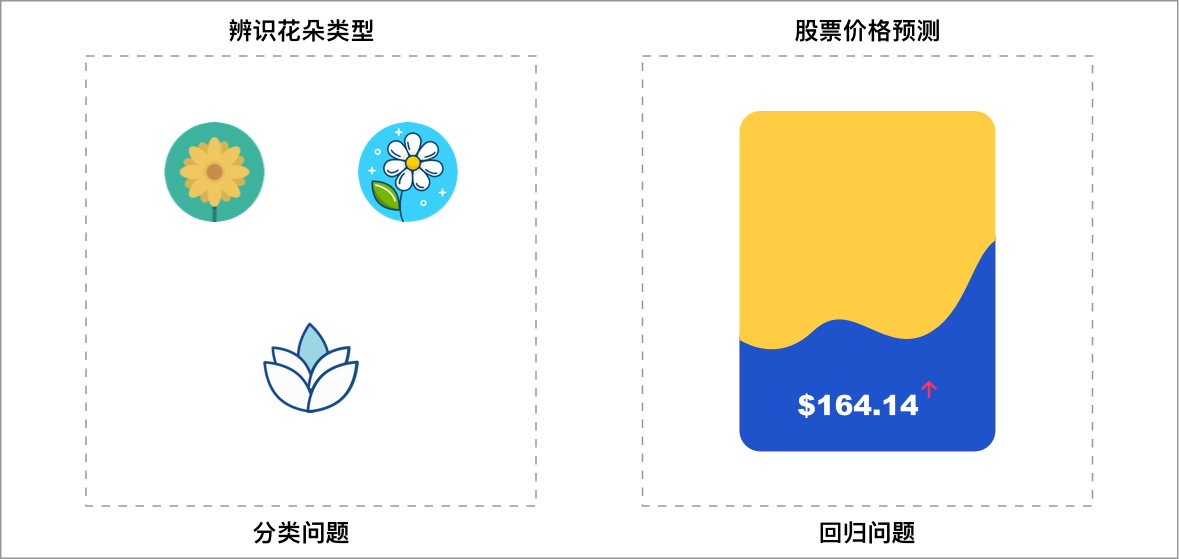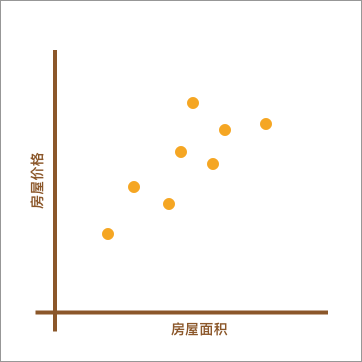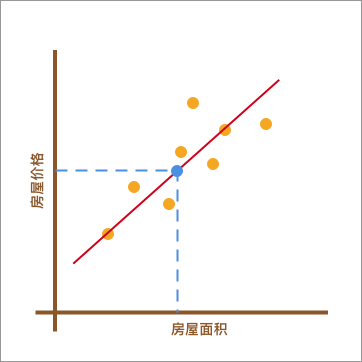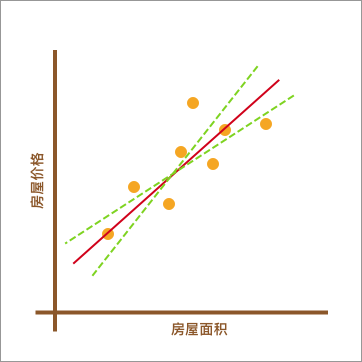## 线性回归原理及实现

### 一元线性回归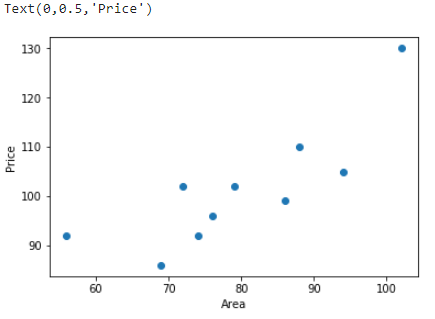$$y(x, w) = w_0 + w_1x \tag{1}$$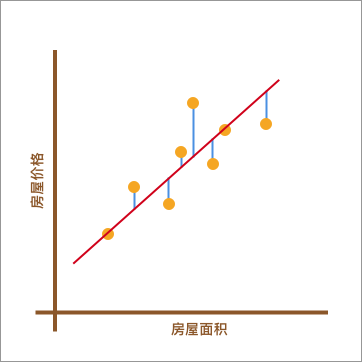### 平方损失函数

$$y_{i}-(w_0 + w_1x_{i}) \tag2$$

$$\sum\limits_{i = 1}^n (y_{i}-(w_0 + w_1x_{i})) \tag3$$

$$\sum\limits_{i = 1}^n (y_{i}-(w_0 + w_1x_{i}))^2\tag4$$

### 最小二乘法及代数求解

$$f = \sum\limits_{i = 1}^n (y_{i}-(w_0 + w_1x_{i}))^2 \tag5$$

$$\frac{\partial f}{\partial w_{0}}=-2(\sum_{i=1}^{n}{y_i}-nw_{0}-w_{1}\sum_{i=1}^{n}{x_i})\\ \frac{\partial f}{\partial w_{1}}=-2(\sum_{i=1}^{n}{x_iy_i}-w_{0}\sum_{i=1}^{n}{x_i}-w_{1}\sum_{i=1}^{n}{x_i}^2) \tag6$$

$$w_{1}=\frac {n\sum_{}^{}{x_iy_i}-\sum_{}^{}{x_i}\sum_{}^{}{y_i}} {n\sum_{}^{}{x_i}^2-(\sum_{}^{}{x_i})^2}\\ w_{0}=\frac {\sum_{}^{}{x_i}^2\sum_{}^{}{y_i}-\sum_{}^{}{x_i}\sum_{}^{}{x_iy_i}} {n\sum_{}^{}{x_i}^2-(\sum_{}^{}{x_i})^2}\tag7$$

### 线性回归 Python 实现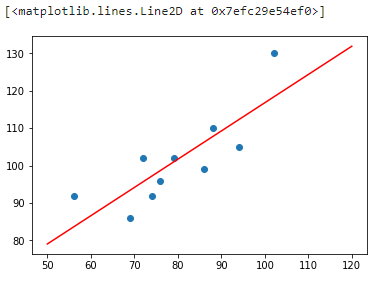### 线性回归 scikit-learn 实现

• fit_intercept: 默认为 True，计算截距项。
• normalize: 默认为 False，不针对数据进行标准化处理。
• copy_X: 默认为 True，即使用数据的副本进行操作，防止影响原数据。
• n_jobs: 计算时的作业数量。默认为 1，若为 -1 则使用全部 CPU 参与运算。

## 最小二乘法的矩阵推导及实现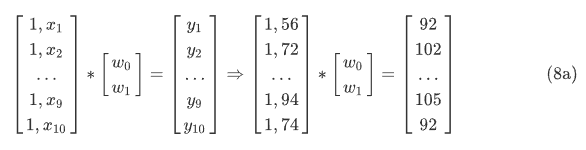（8）式中，W 为，而 X 则是$$f = \sum\limits_{i = 1}^n (y_{i}-(w_0 + w_1x_{i}))^2 =(y-XW)^T(y-XW)\tag{9}$$$$W=(X^TX)^{-1}X^Ty \tag{11}$$